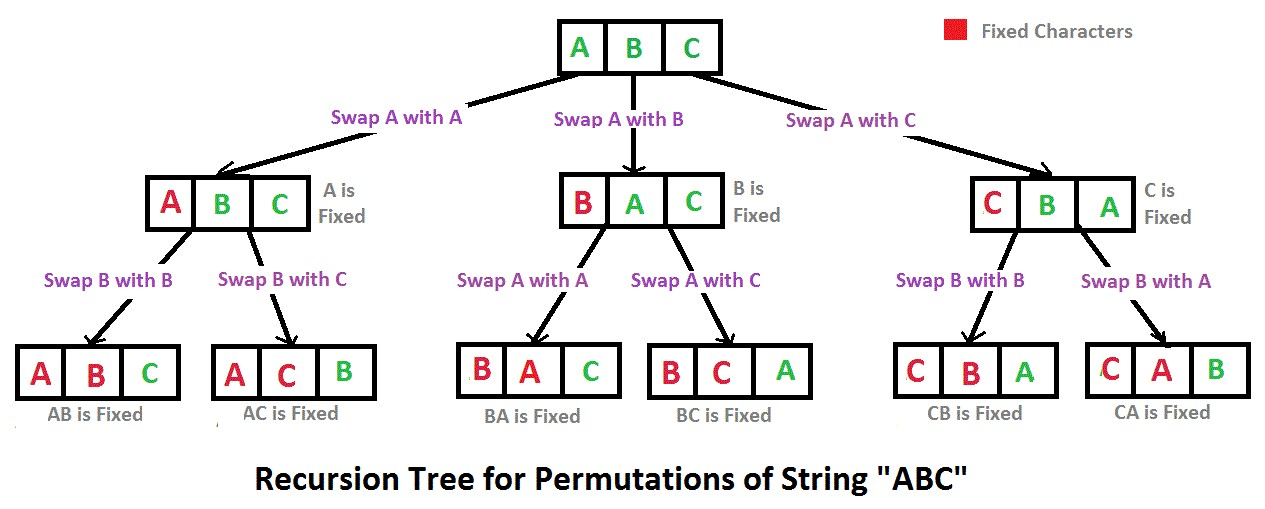# Java打印字符串中字符的所有排列的实现和思路分享

KLQ 2020-04-16 14:57:31 java常见问答 8663

```public ArrayList < String > Permutation(String str)
{
ArrayList < String > list = new ArrayList < String > ();
if (str != null && str.length() > 0)
{
PermutationHelper(str.toCharArray(), 0, list);
Collections.sort(list);
}
return list;
}
private void PermutationHelper(char[] chars, int i, ArrayList < String > list)
{
if (i == chars.length - 1)
{
}
else
{
Set < Character > charSet = new HashSet < Character > ();
for (int j = i; j < chars.length; ++j)
{
if (j == i || !charSet.contains(chars[j]))
{
swap(chars, i, j);
PermutationHelper(chars, i + 1, list);
swap(chars, j, i);
}
}
}
}
private void swap(char[] cs, int i, int j)
{
char temp = cs[i];
cs[i] = cs[j];
cs[j] = temp;
}```

1—9的排列最前面的是123456789，最后面的987654321，

1，从尾部往前找第一个P(i-1) < P(i)的位置

3 4 6 <- 9 <- 8 <- 7 <- 5 <- 2 <- 1

2，从i位置往后找到最后一个大于6的数

3 4 6 -> 9 -> 8 -> 7 5 2 1

3，交换位置i-1和m的值

3 4 7 9 8 6 5 2 1

4，倒序i位置后的所有数据

3 4 7 1 2 5 6 8 9

```public ArrayList < String > Permutation2(String str)
{
ArrayList < String > list = new ArrayList < String > ();
if (str == null || str.length() == 0)
{
return list;
}
char[] chars = str.toCharArray();
Arrays.sort(chars);
int len = chars.length;
while (true)
{
int lIndex = len - 1;
int rIndex;
while (lIndex >= 1 && chars[lIndex - 1] >= chars[lIndex])
{
lIndex--;
}
if (lIndex == 0)
break;
rIndex = lIndex;
while (rIndex < len && chars[rIndex] > chars[lIndex - 1])
{
rIndex++;
}
swap(chars, lIndex - 1, rIndex - 1);
reverse(chars, lIndex);
}
return list;
}
private void reverse(char[] chars, int k)
{
if (chars == null || chars.length <= k)
return;
int len = chars.length;
for (int i = 0; i < (len - k) / 2; i++)
{
int m = k + i;
int n = len - 1 - i;
if (m <= n)
{
swap(chars, m, n);
}
}
}``````import java.util.List;
import java.util.Collections;
import java.util.ArrayList;
public class Solution
{
public static void main(String[] args)
{
Solution p = new Solution();
System.out.println(p.Permutation("abc")
.toString());
}
public ArrayList < String > Permutation(String str)
{
List < String > res = new ArrayList < > ();
if (str != null && str.length() > 0)
{
PermutationHelper(str.toCharArray(), 0, res);
Collections.sort(res);
}
return (ArrayList) res;
}
public void PermutationHelper(char[] cs, int i, List < String > list)
{
if (i == cs.length - 1)
{
String val = String.valueOf(cs);
if (!list.contains(val))
}
else
{
for (int j = i; j < cs.length; j++)
{
swap(cs, i, j);
PermutationHelper(cs, i + 1, list);
swap(cs, i, j);
}
}
}
public void swap(char[] cs, int i, int j)
{
char temp = cs[i];
cs[i] = cs[j];
cs[j] = temp;
}
}```